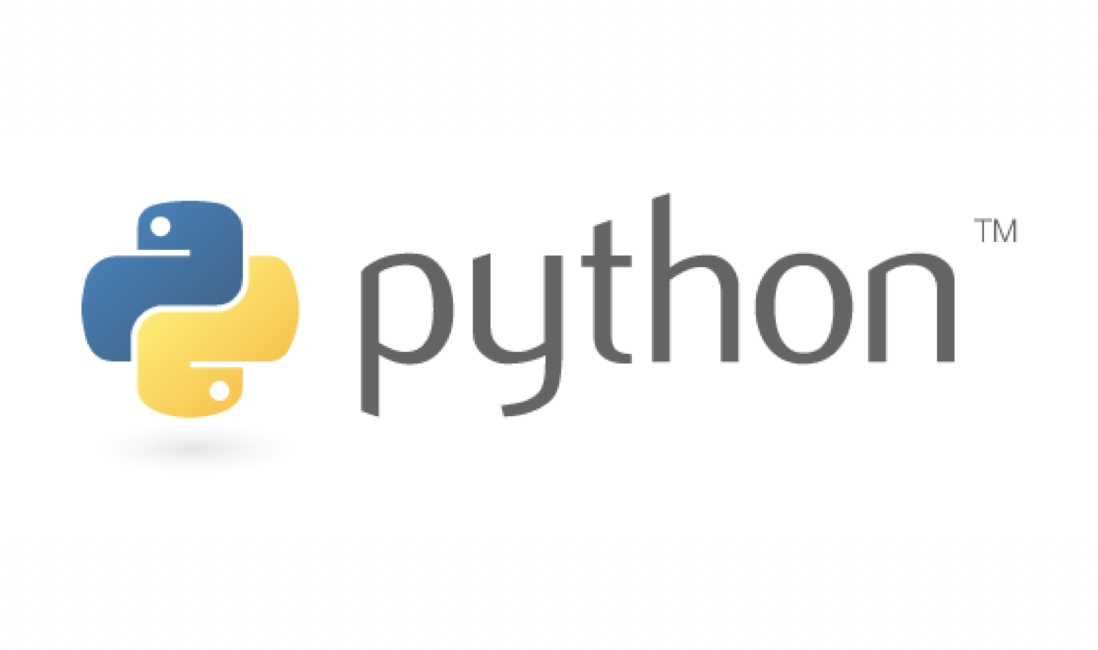Python小技巧 - Python 类型注释Python小技巧 - Python 类型注释

Python 类型注释

Python 3.5+ 开始支持 类型注释 ，以后也可以写一些静态类型的代码了

def add(x: int, y: int) -> int:
return x + y

def sub(x: int, y: int) -> int:
return x - y

def multi(x: int, y: int) -> int:
return x * y

def div(x: int, y: int) -> int:
return x / y

def splice(x: str, y: str) -> str:
return x + ' ' + y

print(sub(4, 3))
print(multi(2, 3))
print(div(10, 5))
print(splice('hello', 'world'))

\$ python main.py
3
1
6
2.0
hello world
12